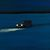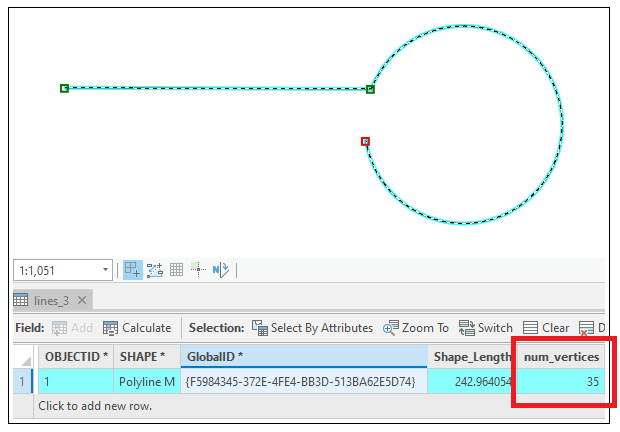# Get the true vertex count (Arcade)

458
0
02-28-2022 06:01 PM
Status: Open
Labels (1)by
Frequent Contributor II

I can get a count of a polyline's vertices by looping through the paths property:

```var line_geo =  Dictionary(Text(Geometry(\$feature)));
var paths = line_geo['paths'];
var vertex_count = 0

for (var path_idx in paths){
for (var vert_idx in paths[path_idx])
{
vertex_count++
}
}
return vertex_count```That code works, but the vertex count is a densified vertex count, which isn't what I want...I was expecting the vertex count to be 3 vertices, not 35 vertices.

Could ESRI give us a way to get the true vertex count? For example, a pointCount() geometry function.

Tags (1)